Let us consider a conductor PQ of length l and area of cross section A. The conductor is placed in a uniform magnetic field of induction B making an angle θ with the field [Fig 3.22].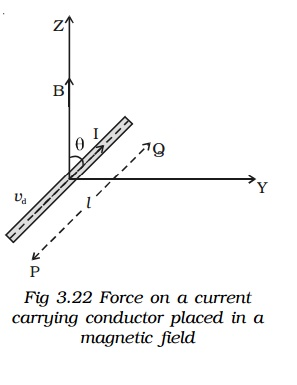A current I flows along PQ. Hence, the electrons are drifted along QP with drift velocity vd. If n is the number of free electrons per unit volume in the conductor, then the current isMultiplying both sides by the length l of the conductor,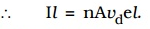Therefore the current element,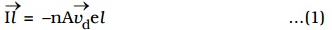The negative sign in the equation indicates that the direction of current is opposite to the direction of drift velocity of the electrons.

Since the electrons move under the influence of magnetic field, the magnetic lorentz force on a moving electron.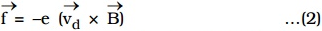The negative sign indicates that the charge of the electron is negative.

The number of free electrons in the conductorThe magnetic Lorentz force on all the moving free electrons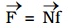Substituting eq (2) and (3) in the above equation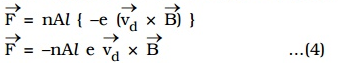Substituting eq (1) in eq (4)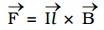This total force on all the moving free electrons is the force on the current carrying conductor placed in the magnetic field.

Magnitude of the force

The magnitude of the force is    F = BIl sin θ

1. If the conductor is placed along the direction of the magnetic field, θ = 0o, Therefore force F = 0.
2. If the conductor is placed perpendicular to the magnetic field, = 90o, F = BIl. Therefore the conductor experiences maximum force.

Direction of force

The direction of the force on a current carrying conductor placed in a magnetic field is given by Fleming’s Left Hand Rule.

The forefinger, the middle finger and the thumb of the left hand are stretched in mutually perpendicular directions. If the forefinger points in the direction of the magnetic field, the middle finger points in the direction of the current, then the thumb points in the direction of the force on the conductor.